• 1．熟悉傅立叶变换的各种性质 2．熟悉基本信号的频域转换 3．熟悉应用FFT对典型信号进行频谱分析的方法 4．熟悉在已经幅频函数|H(jw)|相频函数arg(H(jw)的情况下，利用ifourier函数求傅里叶反变换得到的函数
• matlab傅里叶变换及逆变换快速傅里叶变换，通过编程实战掌握具体应用。包括matlab傅里叶变换、matlab傅里叶逆变换、matlab快速傅里叶变换matlab matlab安装教程 matlab下载 matlab图像处理
• 傅里叶变换傅里叶变换傅里叶变换傅里叶变换傅里叶变换傅里叶变换傅里叶变换傅里叶变换傅里叶变换傅里叶变换傅里叶变换傅里叶变换
• 离散傅里叶变换和快速傅里叶变换，希望对大家有帮助
• 离散傅里叶变换 离散傅里叶变换
离散傅里叶变换
离散傅里叶反变换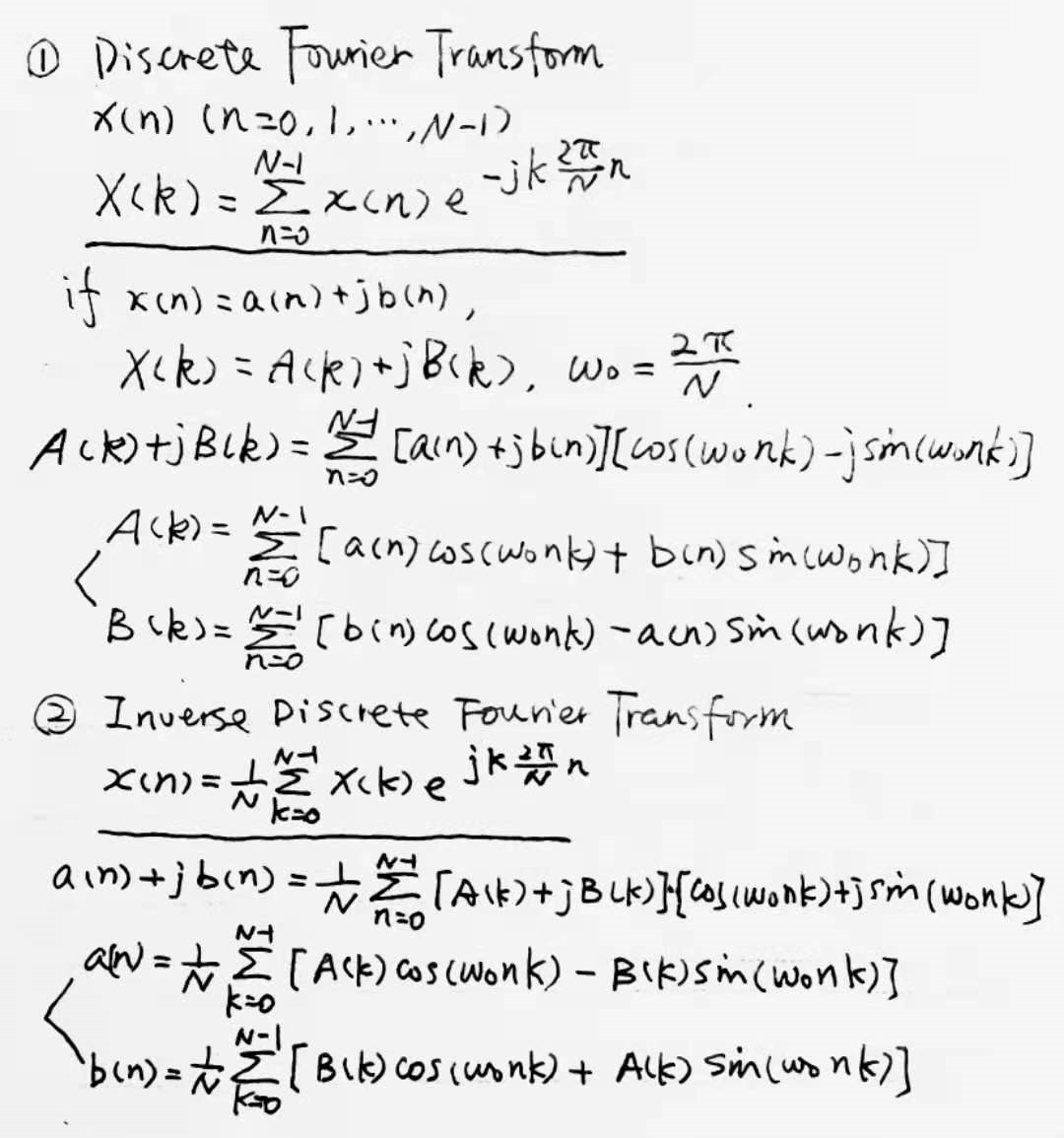傅里叶变换理论值过程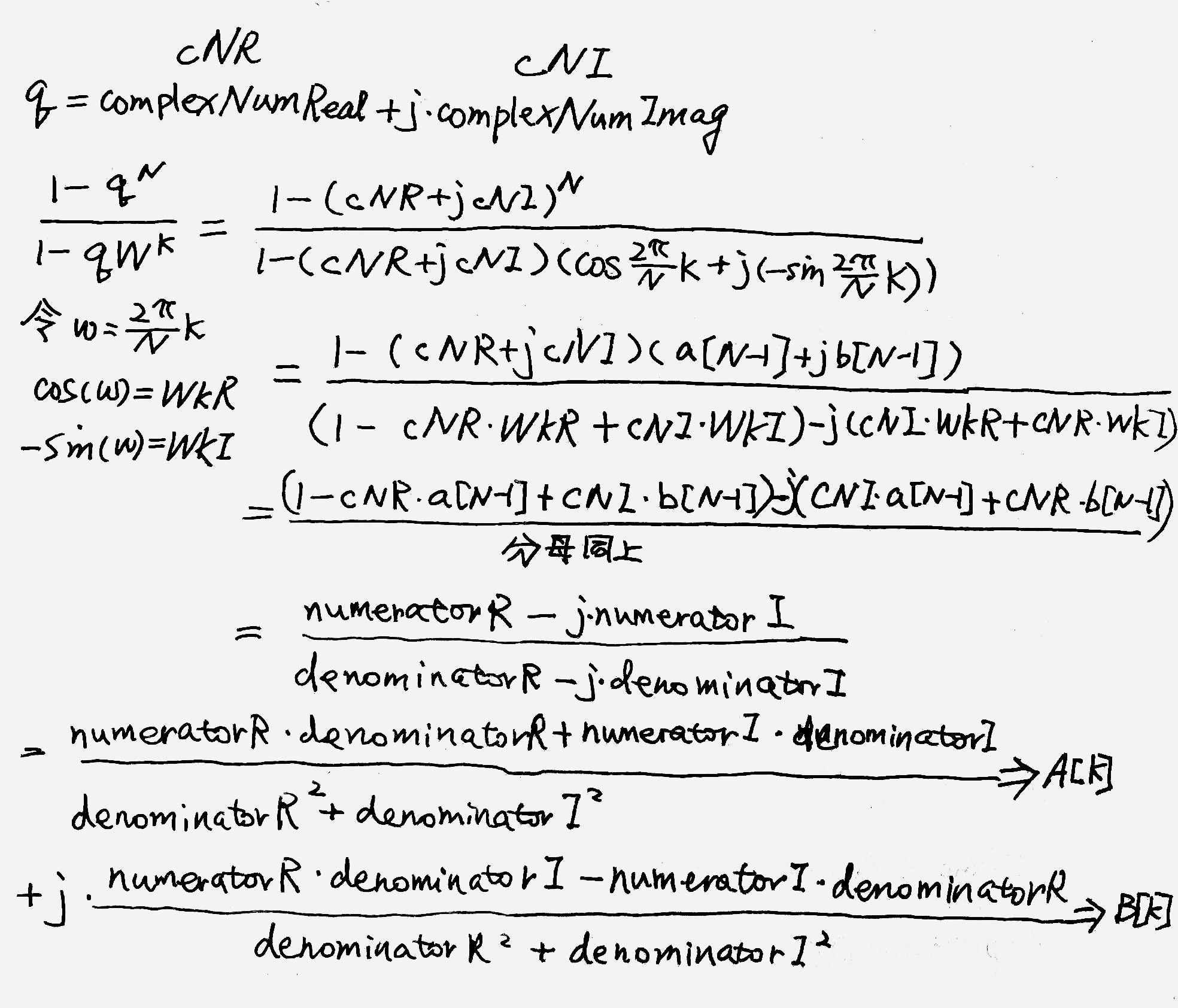dft.c
#include "math.h"
double dft(a,b,A,B,N)
int N;
double a[],b[],A[],B[];
{
double PI=acos(-1.0);
int n,k;
double c,d,w0,w,s;
w0=2*PI/N;
for(k=0;k<N;k++){
w=k*w0;
A[k]=B[k]=0.0;
for(n=0;n<N;n++){
d=n*w;
c=cos(d);
s=sin(d);
A[k]+=c*a[n]+s*b[n];
B[k]+=c*b[n]-s*a[n];
}
}
return PI;
}

idft.c
#include "math.h"
void idft(A,B,a,b,N)
int N;
double A[],B[],a[],b[];
{
double PI=acos(-1.0);
int n,k;
double c,d,w0,w,s;
w0=2*PI/N;
for(n=0;n<N;n++){
w=n*w0;
a[n]=b[n]=0.0;
for(k=0;k<N;k++){
d=k*w;
c=cos(d);
s=sin(d);
a[n]+=(c*A[k]-s*B[k])/N;
b[n]+=(c*B[k]+s*A[k])/N;
}
}
return PI;
}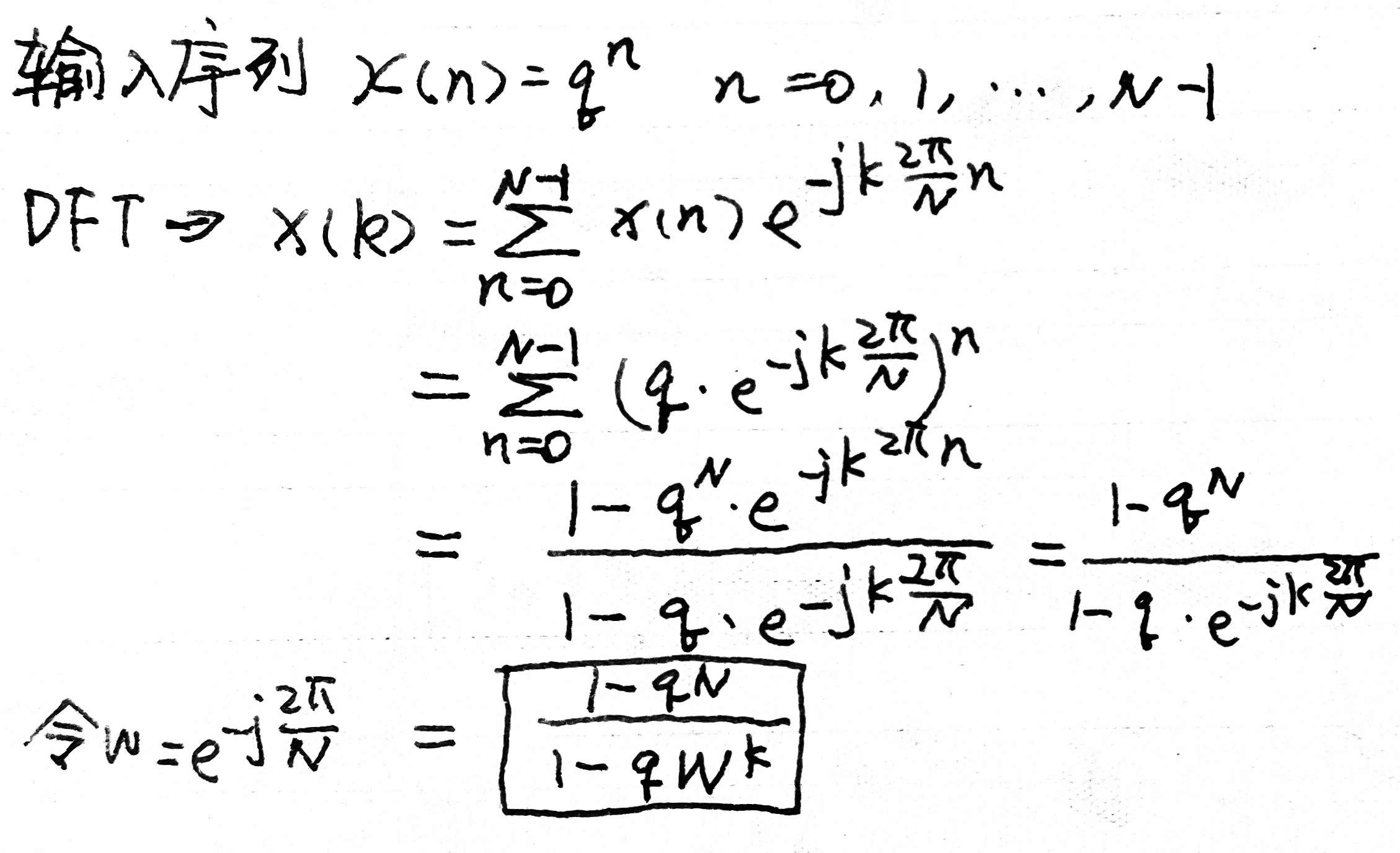其中q=complexNumReal+j*complexNumImaginary
输出x[n]，傅里叶变换实际值，理论值，并且将理论值反变换
main.c
#include "stdio.h"
#include "math.h"
#include "dft.c"
#include "idft.c"
main(){
int i,j,N;
printf("N=");
scanf("%d",&N);
double cNR,cNI;//complexNumReal,complexNumImaginary
double a[N],b[N],A[N],B[N];
printf("complexNumReal=");
scanf("%lf",&cNR);
printf("complexNumImaginary=");
scanf("%lf",&cNI);
a=1.0;
b=0.0;
for(i=1;i<N;i++){
a[i]=cNR*a[i-1]-cNI*b[i-1];
b[i]=cNI*a[i-1]+cNR*b[i-1];
}
printf("----Original Sequence----\n");
for(i=0;i<N/2;i++){
for(j=0;j<2;j++)
printf("|%d:\t%10.6f + j%10.6f|\t",2*i+j+1,a[2*i+j],b[2*i+j]);
printf("\n");
}

double PI=dft(a,b,A,B,N);
printf("----Discrete Fourier Transform----\n");
for(i=0;i<N/2;i++){
for(j=0;j<2;j++)
printf("|%d:\t%10.6f + j%10.6f |\t",2*i+j+1,A[2*i+j],B[2*i+j]);
printf("\n");
}

double nR,nI;//numeratorR,numeratorI
double dR,dI;//denominatorR,denominatorI
double w,WkR,WkI;
printf("----Theoretical Discrete Fourier Transform----\n");
for(int k=0;k<N;k++){
w=(2*PI/N)*k;   //W^k=e^-j*k*(2*PI/N)=cos(w)+j*(-1)*sin(w)
WkR=cos(w);
WkI=(-1)*sin(w);
nR=1-cNR*a[N-1]+cNI*b[N-1];
nI=cNI*a[N-1]+cNR*b[N-1];
dR=1-cNR*WkR+cNI*WkI;
dI=cNI*WkR+cNR*WkI;
A[k]=(nR*dR+nI*dI)/(pow(dR,2)+pow(dI,2));
B[k]=(nR*dI-nI*dR)/(pow(dR,2)+pow(dI,2));
}
for(i=0;i<N/2;i++){
for(j=0;j<2;j++)
printf("|%d:\t%10.6f + j%10.6f |\t",2*i+j+1,A[2*i+j],B[2*i+j]);
printf("\n");
}

idft(A,B,a,b,N);
printf("****Inverse Discrete Fourier Transform****\n");
for(i=0;i<N/2;i++){
for(j=0;j<2;j++)
printf("|%d:\t%10.6f + j%10.6f |\t",2*i+j+1,a[2*i+j],b[2*i+j]);
printf("\n");
}
}



展开全文c语言 信号处理 idft
• 傅里叶变换和傅里叶逆变换的C++代码，亲测，有详细的解释。 有问题可以留言。
• 精通matlab傅里叶变换及逆变换快速傅里叶变换matlab 编程语言 Matlab
• 傅里叶变换和快速傅里叶变换VC++算法实现演示 VC++6编译, MFC程序
• 傅里叶变换和傅里叶级数之间的区别与联系，详细推导个人理解。我的CSDN博客中也有这一篇文章。
• 基于matlab的傅里叶变换变换的标准形式。
• 离散傅里叶变换离散傅里叶变换离散傅里叶变换离散傅里叶变换离散傅里叶变换离散傅里叶变换离散傅里叶变换离散傅里叶变换离散傅里叶变换离散傅里叶变换离散傅里叶变换离散傅里叶变换离散傅里叶变换离散傅里叶变换
• ## OpenCV中的图像变换——傅里叶变换

万次阅读 多人点赞 2021-07-22 20:11:24
这篇博客将介绍OpenCV中的图像变换，包括用Numpy、OpenCV计算图像的傅里叶变换，以及傅里叶变换的一些应用；
OpenCV中的图像变换——傅里叶变换1. 效果图2. 原理3. 源码3.1 Numpy实现傅里叶变换3.2 OpenCV实现傅里叶变换3.3 HPF or LPF？参考
这篇博客将介绍OpenCV中的图像变换，包括用Numpy、OpenCV计算图像的傅里叶变换，以及傅里叶变换的一些应用；

2D Discrete Fourier Transform (DFT)二维离散傅里叶变换
Fast Fourier Transform (FFT) 快速傅里叶变换

傅立叶变换用于分析各种滤波器的频率特性。对于图像采用二维离散傅立叶变换（DFT）求频域。一种称为快速傅立叶变换（FFT）的快速算法用于DFT的计算。

OpenCV使用cv2.dft()、cv2.idft() 实现傅里叶变换，效率更高一些（比OpenCV快3倍）
Numpy使用np.ifft2() 、np.fft.ifftshift() 实现傅里叶变换，使用更友好一些；

1. 效果图
灰度图 VS 傅里叶变换效果图如下：
可以看到白色区域大多在中心，显示低频率的内容比较多。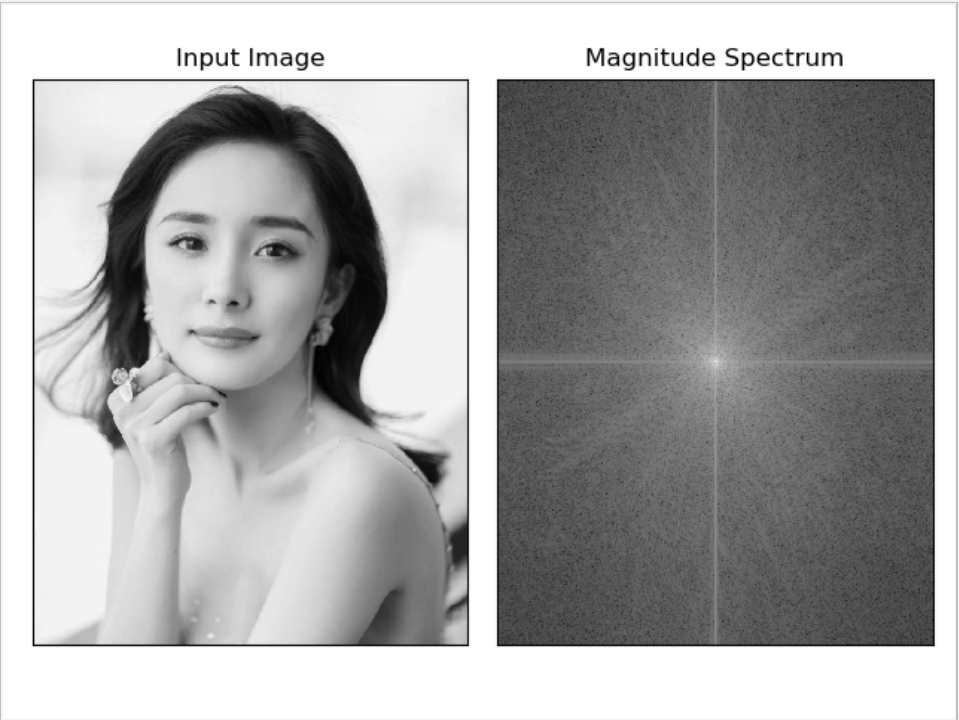傅里叶变换去掉低频内容后效果图如下：
可以看到使用矩形滤波后，效果并不好，有波纹的振铃效果；用高斯滤波能好点；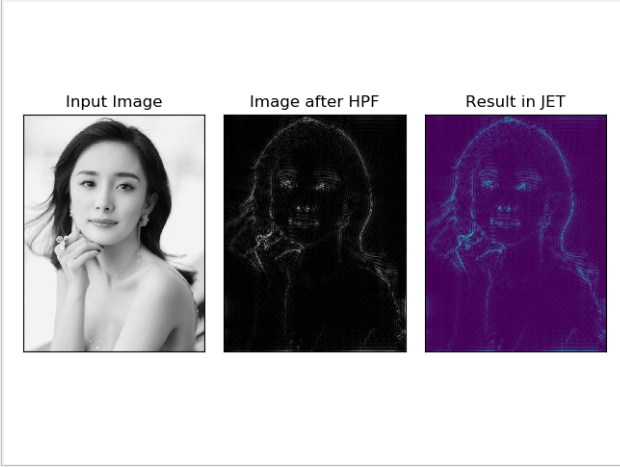傅里叶变换去掉高频内容后效果图如下：
删除图像中的高频内容，即将LPF应用于图像，它实际上模糊了图像。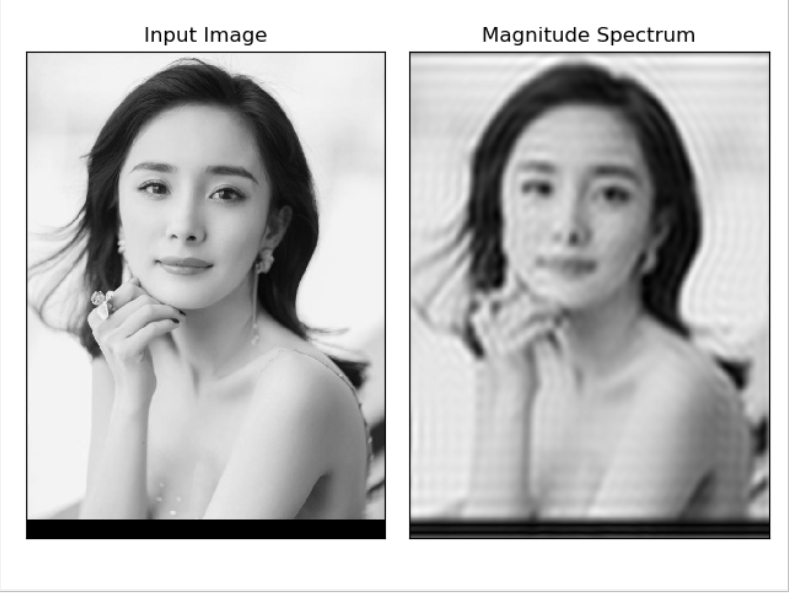各滤波器是 HPF（High Pass Filter）还是 LPF（Low Pass Filter），一目了然：
拉普拉斯是高频滤波器；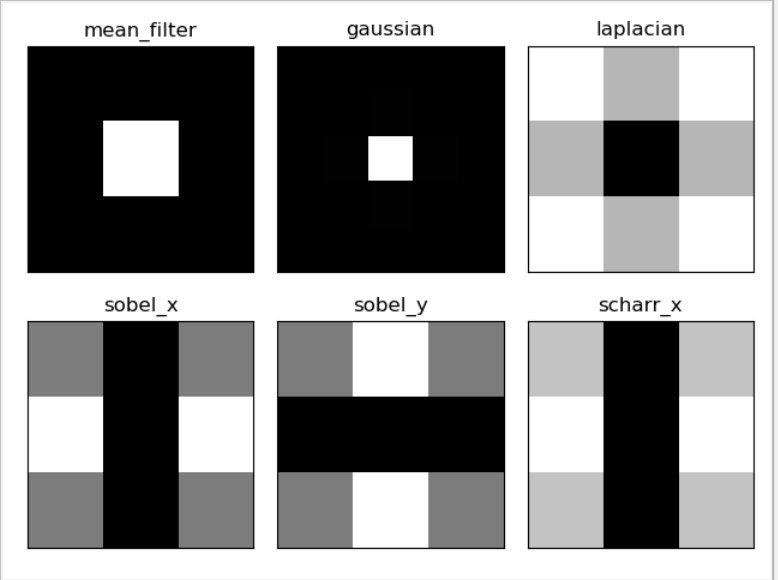2. 原理

DFT的性能优化：在一定的阵列尺寸下，DFT计算的性能较好。当数组大小为2的幂时，速度最快。大小为2、3和5的乘积的数组也可以非常有效地处理。

为达到最佳性能，可以通过OpenCV提供的函数cv2.getOptimalDFTSize() 寻找最佳尺寸。
然后将图像填充成最佳性能大小的阵列，对于OpenCV，必须手动填充零。但是对于Numpy，可以指定FFT计算的新大小,会自动填充零。
通过使用最优阵列，基本能提升4倍的效率。而OpenCV本身比Numpy效率快近3倍；

拉普拉斯是高通滤波器（High Pass Filter）

3. 源码
3.1 Numpy实现傅里叶变换
# 傅里叶变换

import cv2
import numpy as np
from matplotlib import pyplot as plt

# 使用Numpy实现傅里叶变换：fft包
# fft.fft2() 进行频率变换
# 参数1：输入图像的灰度图
# 参数2：>输入图像 用0填充；  <输入图像 剪切输入图像； 不传递 返回输入图像
f = np.fft.fft2(img)

# 一旦得到结果，零频率分量（直流分量）将出现在左上角。
# 如果要将其置于中心，则需要使用np.fft.fftshift()将结果在两个方向上移动。
# 一旦找到了频率变换，就能找到幅度谱。
fshift = np.fft.fftshift(f)
magnitude_spectrum = 20 * np.log(np.abs(fshift))

plt.subplot(121), plt.imshow(img, cmap='gray')
plt.title('Input Image'), plt.xticks([]), plt.yticks([])
plt.subplot(122), plt.imshow(magnitude_spectrum, cmap='gray')
plt.title('Magnitude Spectrum'), plt.xticks([]), plt.yticks([])
plt.show()

# 找到了频率变换，就可以进行高通滤波和重建图像，也就是求逆DFT
rows, cols = img.shape
crow, ccol = rows // 2, cols // 2
fshift[crow - 30:crow + 30, ccol - 30:ccol + 30] = 0
f_ishift = np.fft.ifftshift(fshift)
img_back = np.fft.ifft2(f_ishift)
img_back = np.abs(img_back)

# 图像渐变章节学习到：高通滤波是一种边缘检测操作。这也表明大部分图像数据存在于频谱的低频区域。
# 仔细观察结果可以看到最后一张用JET颜色显示的图像，有一些瑕疵（它显示了一些波纹状的结构，这就是所谓的振铃效应。）
plt.subplot(131), plt.imshow(img, cmap='gray')
plt.title('Input Image'), plt.xticks([]), plt.yticks([])
plt.subplot(132), plt.imshow(img_back, cmap='gray')
plt.title('Image after HPF'), plt.xticks([]), plt.yticks([])
plt.subplot(133), plt.imshow(img_back)
plt.title('Result in JET'), plt.xticks([]), plt.yticks([])

plt.show()

3.2 OpenCV实现傅里叶变换
import cv2
import numpy as np
from matplotlib import pyplot as plt

rows, cols = img.shape
print(rows, cols)

# 计算DFT效率最佳的尺寸
nrows = cv2.getOptimalDFTSize(rows)
ncols = cv2.getOptimalDFTSize(cols)
print(nrows, ncols)

nimg = np.zeros((nrows, ncols))
nimg[:rows, :cols] = img
img = nimg

# OpenCV计算快速傅里叶变换，输入图像应首先转换为np.float32，然后使用函数cv2.dft()和cv2.idft()。
# 返回结果与Numpy相同，但有两个通道。第一个通道为有结果的实部，第二个通道为有结果的虚部。
dft = cv2.dft(np.float32(img), flags=cv2.DFT_COMPLEX_OUTPUT)
dft_shift = np.fft.fftshift(dft)

magnitude_spectrum = 20 * np.log(cv2.magnitude(dft_shift[:, :, 0], dft_shift[:, :, 1]))

plt.subplot(121), plt.imshow(img, cmap='gray')
plt.title('Input Image'), plt.xticks([]), plt.yticks([])
plt.subplot(122), plt.imshow(magnitude_spectrum, cmap='gray')
plt.title('Magnitude Spectrum'), plt.xticks([]), plt.yticks([])
plt.show()

rows, cols = img.shape
crow, ccol = rows // 2, cols // 2

# 如何删除图像中的高频内容，即我们将LPF应用于图像。它实际上模糊了图像。
# 为此首先创建一个在低频时具有高值的掩码，即传递LF内容，在HF区域为0。
mask = np.zeros((rows, cols, 2), np.uint8)
mask[crow - 30:crow + 30, ccol - 30:ccol + 30] = 1

f_ishift = np.fft.ifftshift(fshift)
img_back = cv2.idft(f_ishift)
img_back = cv2.magnitude(img_back[:, :, 0], img_back[:, :, 1])

plt.subplot(121), plt.imshow(img, cmap='gray')
plt.title('Input Image'), plt.xticks([]), plt.yticks([])
plt.subplot(122), plt.imshow(img_back, cmap='gray')
plt.title('Magnitude Spectrum'), plt.xticks([]), plt.yticks([])
plt.show()

3.3 HPF or LPF？
import cv2
import numpy as np
from matplotlib import pyplot as plt

# 简单的均值滤波
mean_filter = np.ones((3, 3))

# 构建高斯滤波
x = cv2.getGaussianKernel(5, 10)
gaussian = x * x.T

# 不同的边缘检测算法Scharr-x方向
scharr = np.array([[-3, 0, 3],
[-10, 0, 10],
[-3, 0, 3]])
# Sobel_x
sobel_x = np.array([[-1, 0, 1],
[-2, 0, 2],
[-1, 0, 1]])
# Sobel_y
sobel_y = np.array([[-1, -2, -1],
[0, 0, 0],
[1, 2, 1]])
# 拉普拉斯
laplacian = np.array([[0, 1, 0],
[1, -4, 1],
[0, 1, 0]])

filters = [mean_filter, gaussian, laplacian, sobel_x, sobel_y, scharr]
filter_name = ['mean_filter', 'gaussian', 'laplacian', 'sobel_x', \
'sobel_y', 'scharr_x']
fft_filters = [np.fft.fft2(x) for x in filters]
fft_shift = [np.fft.fftshift(y) for y in fft_filters]
mag_spectrum = [np.log(np.abs(z) + 1) for z in fft_shift]

for i in range(6):
plt.subplot(2, 3, i + 1), plt.imshow(mag_spectrum[i], cmap='gray')
plt.title(filter_name[i]), plt.xticks([]), plt.yticks([])

plt.show()


参考

https://docs.opencv.org/3.0-beta/doc/py_tutorials/py_imgproc/py_transforms/py_fourier_transform/py_fourier_transform.html#fourier-transform


展开全文python opencv
• 实验二 离散时间傅里叶变换和离散傅里叶变换 一 实验目的 深刻理解离散时间信号傅里叶变换的定义与连续傅里叶变换之间的关系 深刻理解序列频谱的性质连续的周期的等 能用 MATLAB 编程实现序列的 DTFT 并能显示频谱...
• 请问一个10240长的平稳序列，取前400个点做傅里叶变换，取这400个点的频谱再用逆傅里叶变换能还原出10240个点的序列数据么？ xl为10240长的序列 len(xl) = 10240 fr = fft(xl[0:400]) fr逆傅里叶变换能还原...开发语言
• 傅里叶变换.pdf傅里叶变换.pdf傅里叶变换.pdf傅里叶变换.pdf
• 傅里叶变换 快速傅里叶变换 VC++ MFC VC6程序
• 在13期中，我们提到：信号与系统课程的三大变换(傅里叶变换、拉普拉斯变换z变换)中，傅里叶变换无疑是最为重要的变换，而傅里叶变换的性质又是其中的重点。连续时间信号傅里叶变换的性质很多，需要掌握的有：对称...


信号专栏15期。大道至简，心诚则灵！和我们一起轻松学懂信号！在13期中，我们提到：信号与系统课程的三大变换(傅里叶变换、拉普拉斯变换和z变换)中，傅里叶变换无疑是最为重要的变换，而傅里叶变换的性质又是其中的重点。连续时间信号傅里叶变换的性质很多，需要掌握的有：对称性(对偶性、互易对称性)、线性、奇偶性(共轭对称性)、展缩特性(尺度变换特性)、时移特性、频移特性以及频域卷积特性等。每个性质包含三方面的内容：性质本身的数学表示、证明和应用。应用包括在傅里叶变换和反变换计算中的应用，以及理解该性质表达的物理含义。今天介绍傅里叶变换的时移特性和尺度变换特性一、傅里叶变换的时移特性若已知f(t)的傅里叶变换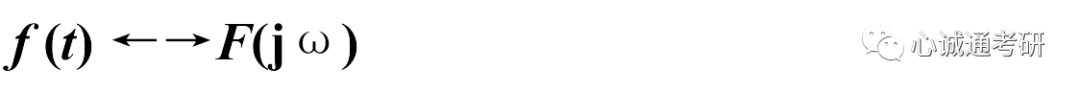那么可知f(t-t0)的傅里叶变换为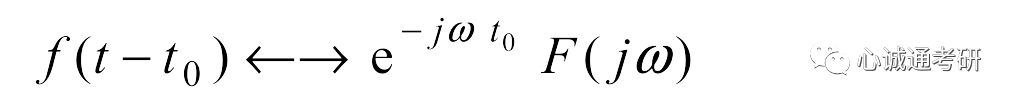二、该性质的证明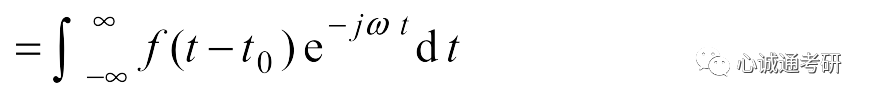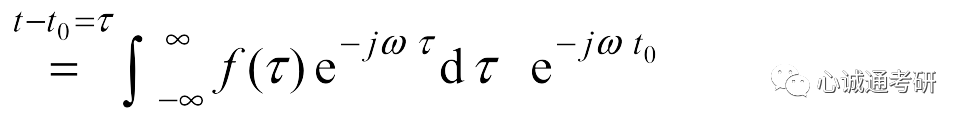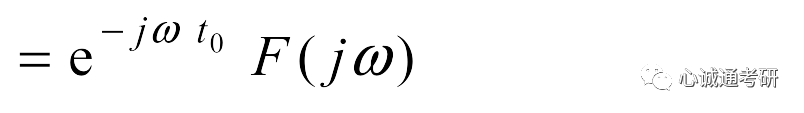三、傅里叶变换的尺度变换特性若已知f(t)的傅里叶变换那么f(at)的傅里叶变换为，此处a为非0实数。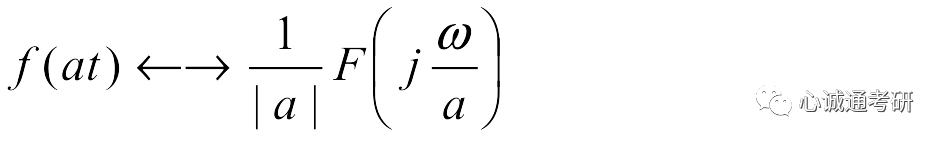四、该性质的证明若a>0，则若a<0，则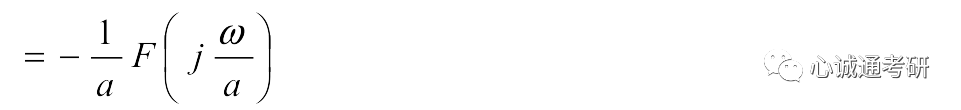两种情况可以合并表示为特别的，当a=-1时五、应用举例实际中，我们遇到的题目可能是多个性质结合在一起的。下面。举一个线性、时移、尺度变换相结合的例子。例：已知f(t)波形如下图所示，求它的傅里叶变换。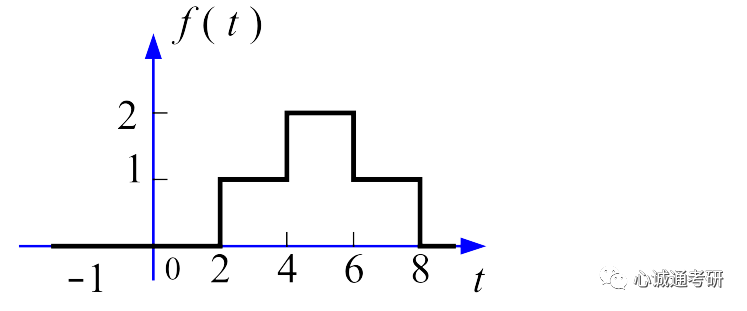f(t)可以分解为下面两个波形相加f1(t)+f2(t)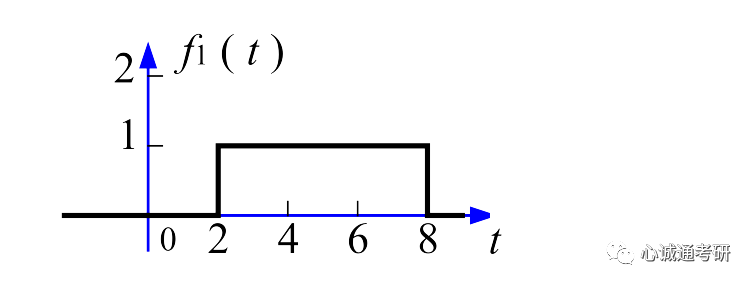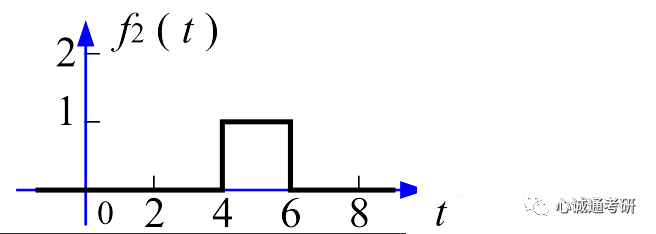该波形是门函数的时移，可以记作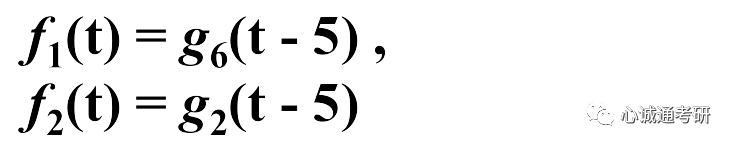根据时移特性可知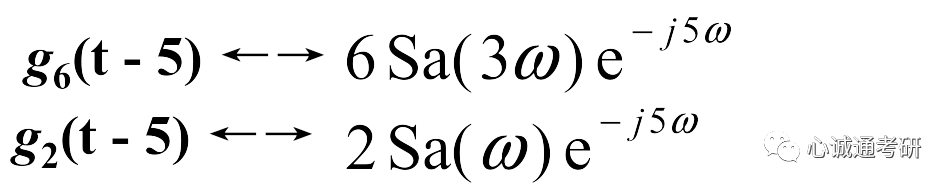根据线性特性，可以得出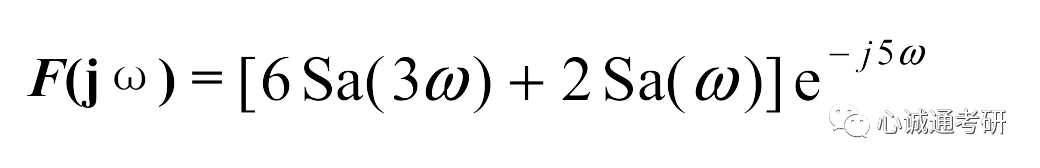在门函数这一步，也可以通过单位矩形脉冲的傅里叶变换的尺度变换来得到两个函数的傅里叶变换，此处不再详述。有啥疑问欢迎加qq群：416076455。点个“在看”，祝你成功上岸！


展开全文• 图2 傅里叶变换来源百度百科 为了让变换系数不为0，在傅里叶级数的基础上乘了T。 2pi*k/T变成了w。在傅里叶级数中乘了T,逆变换时乘1/T。为了凑出dw(一个角速度增量)。再配一个2pi/2pi,变成了一个2pi/(2piT)，即dw/(2...
• 关于傅里叶级数（一般、指数、广义）、傅里叶变换、离散时间傅里叶变换傅里叶变换的一个补充说明 傅里叶级数 傅里叶级数定义式为： 这个定义是我们在大学里学的。你们还记得这个级数什么时候收敛吗？比如说，一...FFT
• 傅里叶变换深入了解傅里叶变换深入了解傅里叶变换深入了解傅里叶变换深入了解
• 3.1 Python图像的频域图像增强-图像的傅里叶变换和反变换 文章目录3.1 Python图像的频域图像增强-图像的傅里叶变换和反变换1 算法原理2 代码3 效果 1 算法原理 图像的傅里叶变换和反变换（需要考虑图像旋转、平移时...opencv python 计算机视觉
• 快速傅里叶变换，FFT，Chirp－Z变换，按频率抽取（DIF）的FTT
• import numpy as np from math import * x=[1,2,3,4,5] ...'''傅里叶变换''' def fft(x): x = np.asarray(x, dtype=float) N = x.shape n = np.arange(N) k = n.reshape((N, 1)) M = np.exp(-2j * n...
• 傅里叶变换 计算傅里叶变换 傅里叶变换 鉴于这种想法，任何信号，当然任何周期性信号，都可以由一系列正弦曲线组成，我们将开始从级数（Series）的概念转向连续信号的概念。我们将要讨论一些东西，这些东西可以让...机器视觉
• 计算信号的傅里叶变换，信号的反傅里叶变换，周期信号的展开，
• ## 傅里叶变换 一维离散傅里叶变换

万次阅读 热门讨论 2019-11-06 21:08:43
DFT:(Discrete Fourier Transform)离散傅里叶变换傅里叶变换在时域频域上都呈离散的形式，将信号的时域采样变换为其DTFT的频域采样。在形式上，变换两端（时域频域上）的序列是有限长的，而实际上这两组序列...IDFT
• 功能描述： ...给定信号，对其进行傅里叶变换然后再进行变换，绘制原始信号、傅里叶变换、傅里叶变换的结果图像。给定信号，滤除其中某个频率范围的信号，绘制图像。 参考代码： 运行结果： ...python
• ## 从傅里叶变换到加窗傅里叶变换

万次阅读 多人点赞 2017-12-10 14:00:49信号与系统 信号处理...